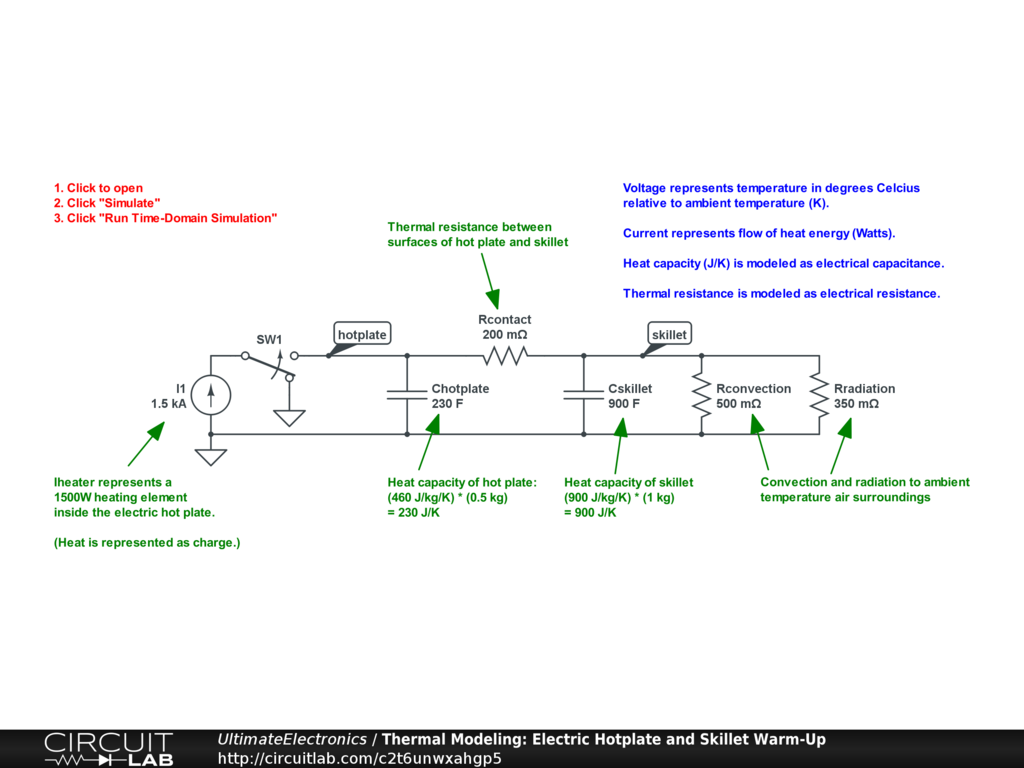Chapter 1 Example Circuits

Interactive Exercise Click any circuit to open in a new tab. Most include simulations.

From Algebraic Approximations:

Plotting Tangent Line Approximation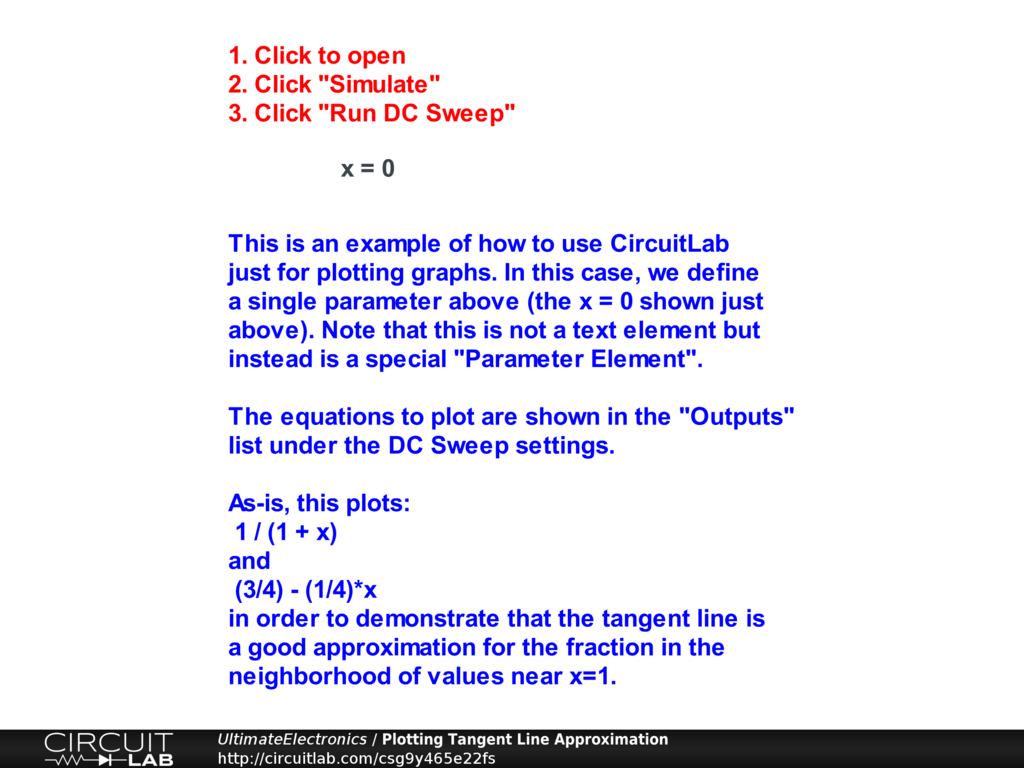Midpoint Tangent, Endpoint Interpolation, and Linear Least Squares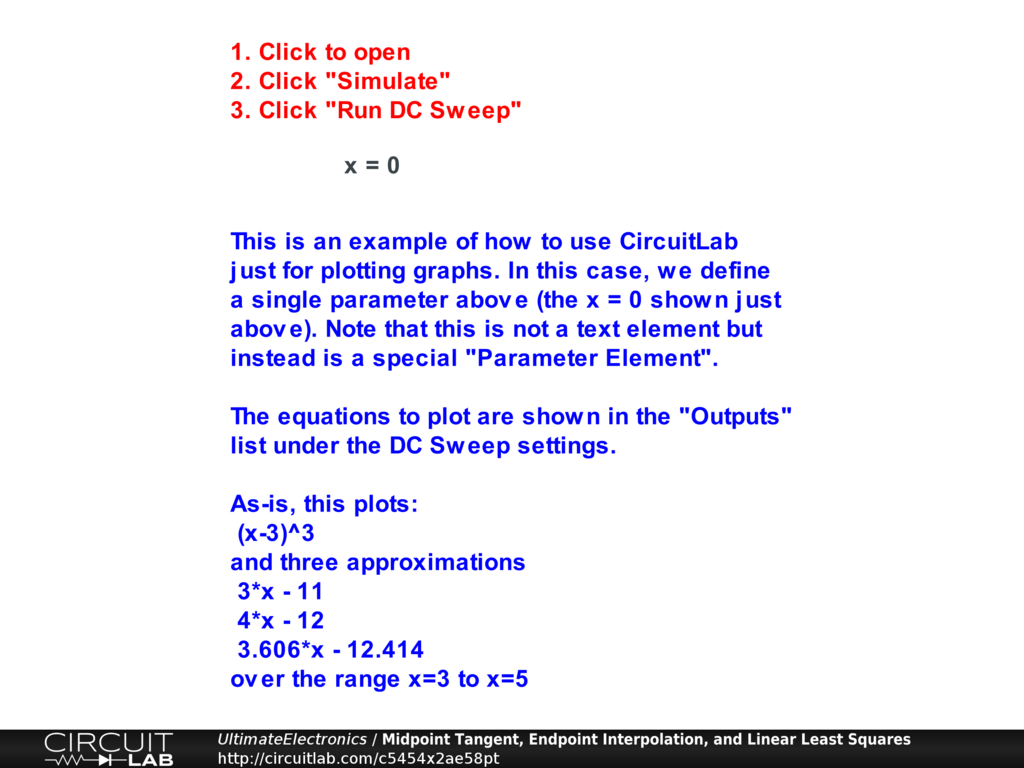From Orders of Magnitude, Logarithmic Scales, and Decibels:

Log-Log Plotting Approximations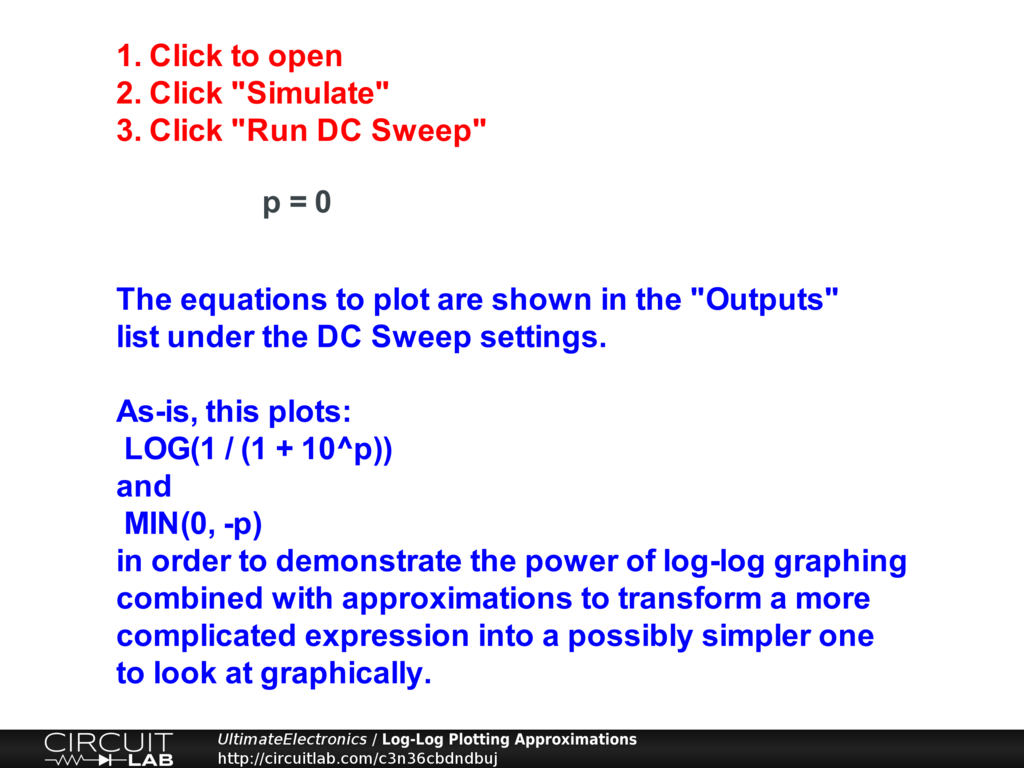From Complex Numbers:

RC Low-Pass Filter as Complex Number Multiplication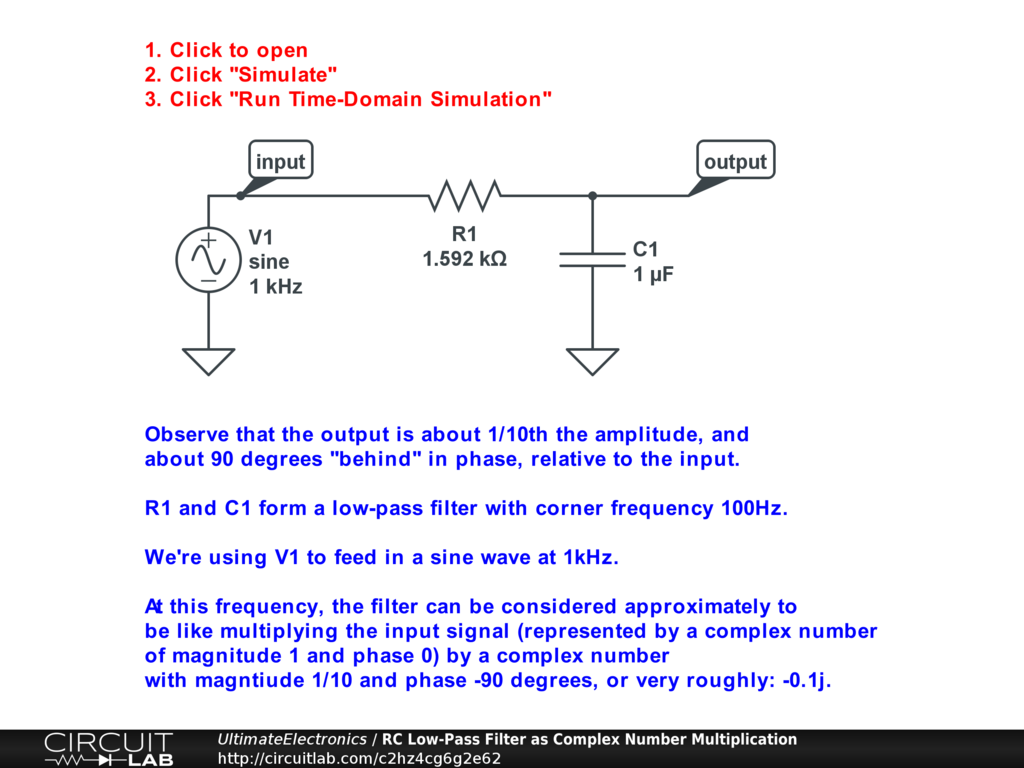From Linear & Nonlinear:

Multiple Linear Equations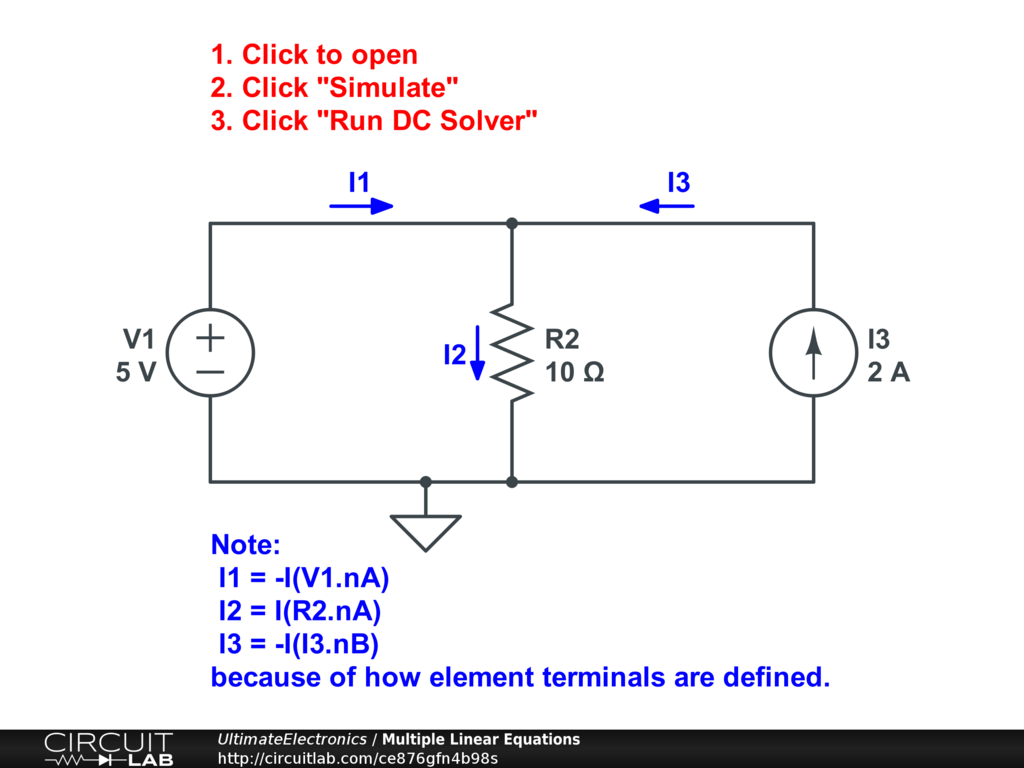Multiple Linear Equations - Frequency Domain Shows Slopes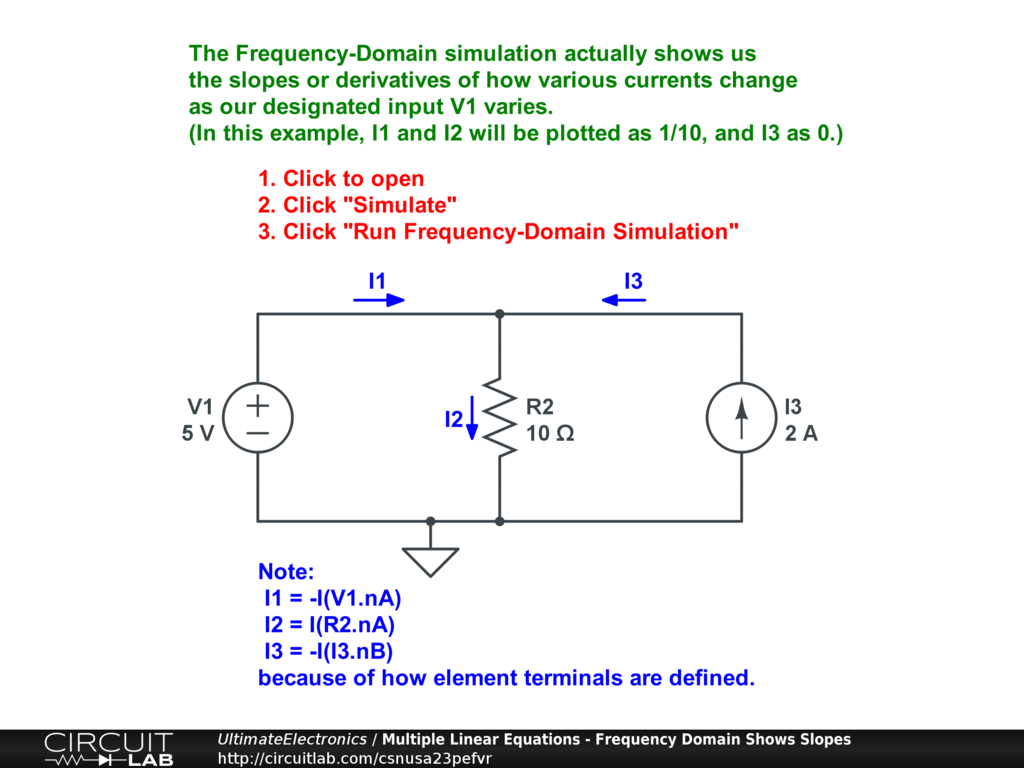Multiple Linear Equations with Sine Wave Input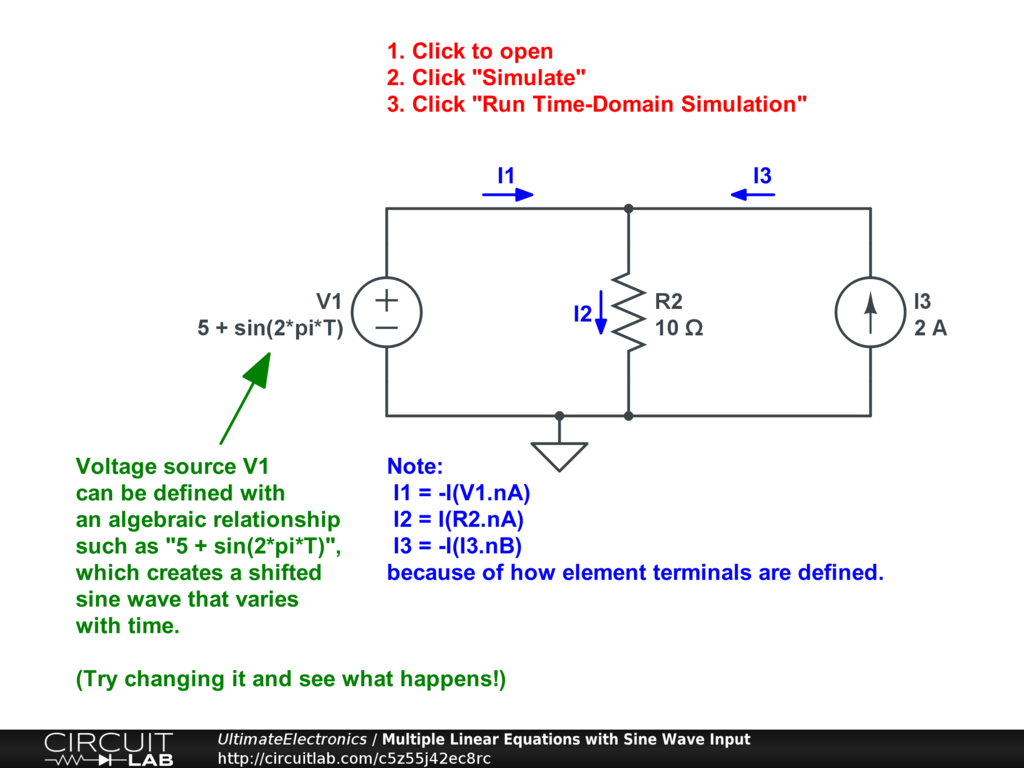Multiple Linear Equations with Two Sine Wave Input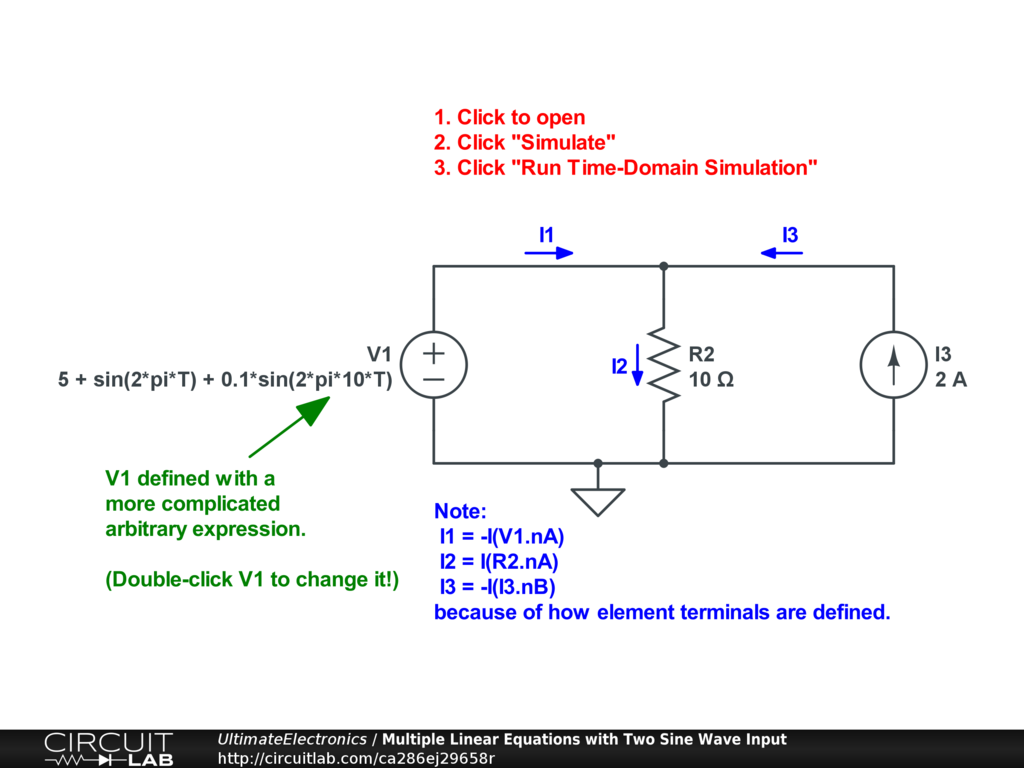Multiple Linear Equations with Multiple Additive Inputs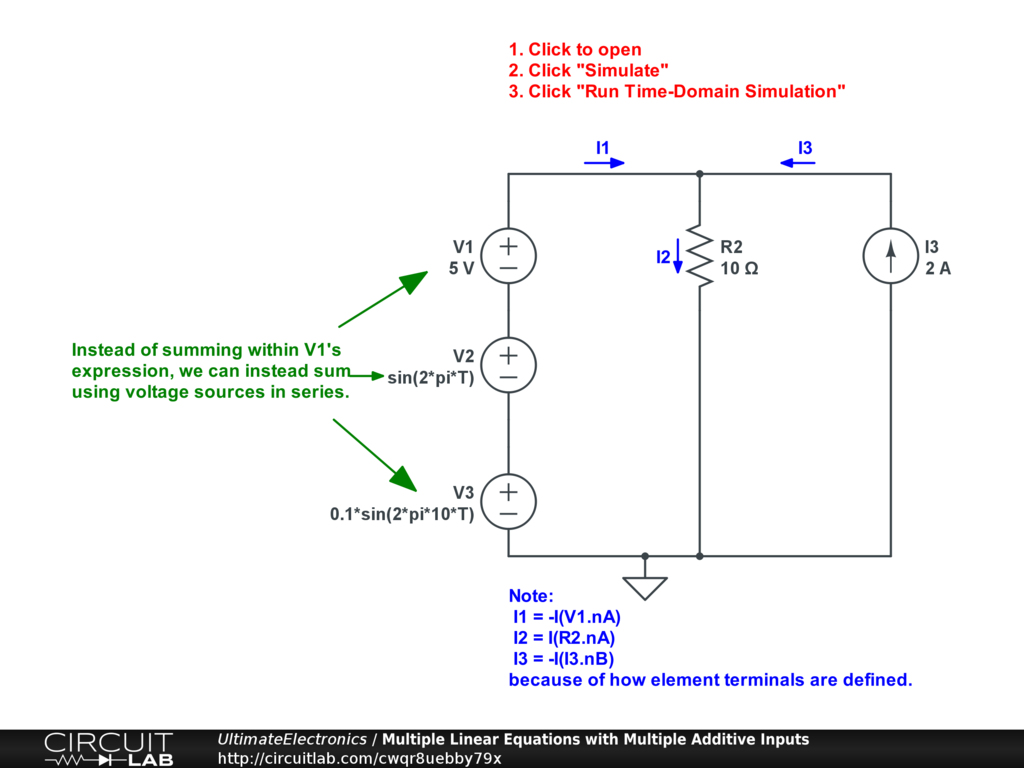Newton-Raphson Method Example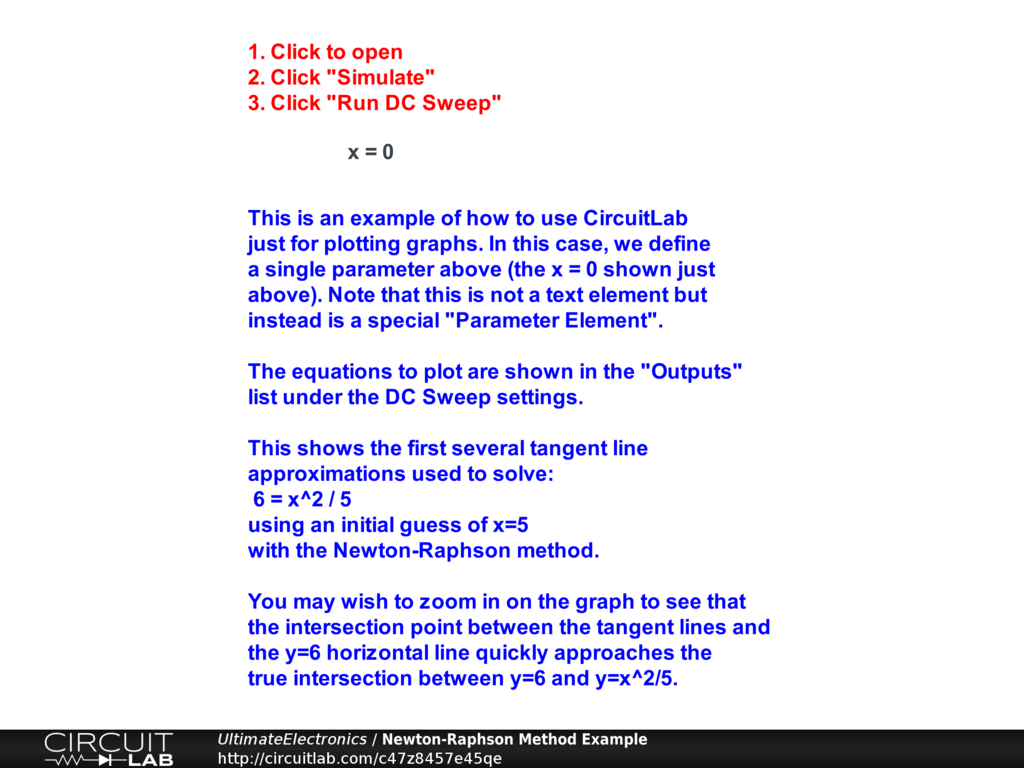555 Adjustable PWM with RC Filtered Output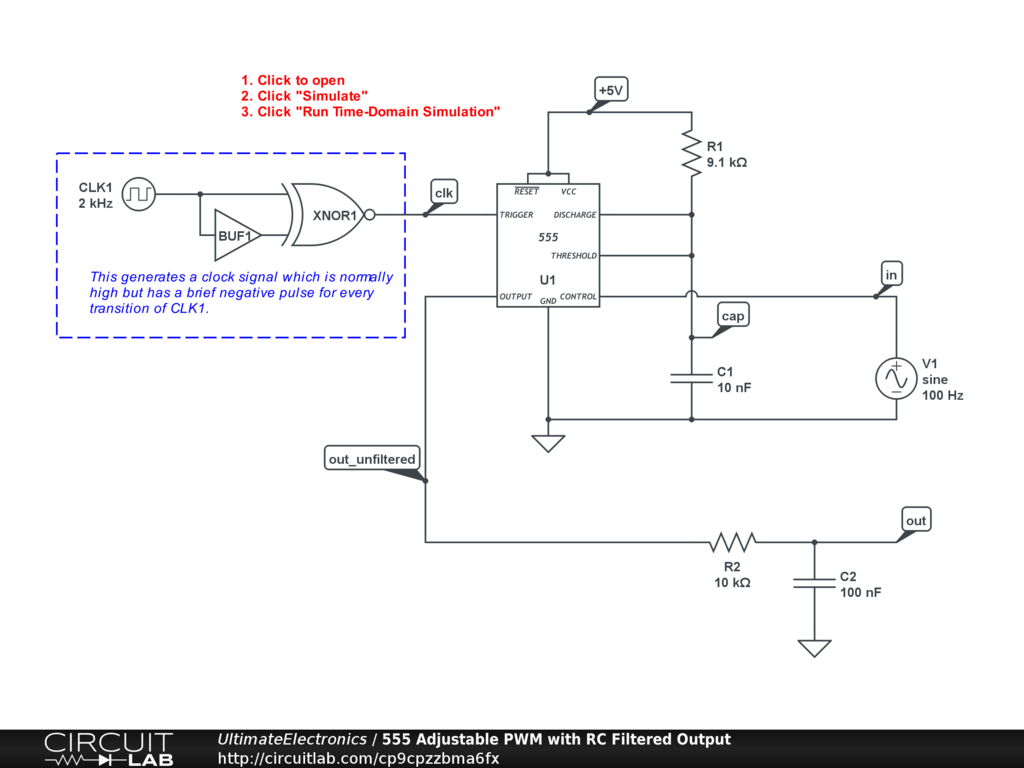555 Voltage Controlled Oscillator (VCO)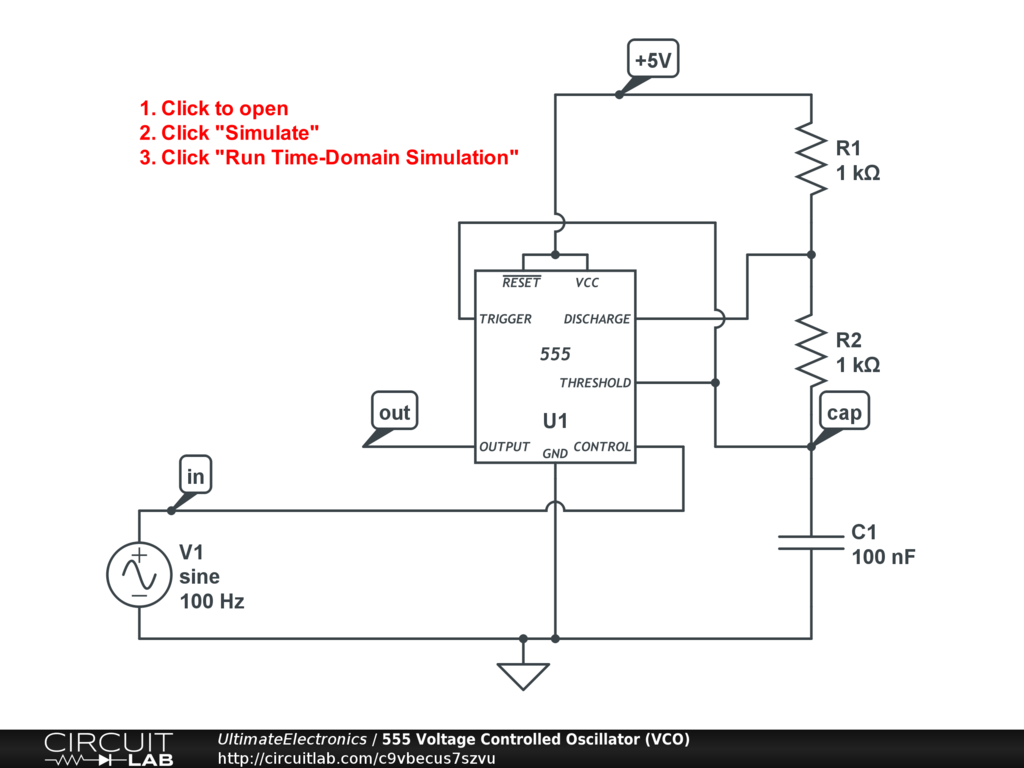From Systems of Equations:

System of Two Linear Equations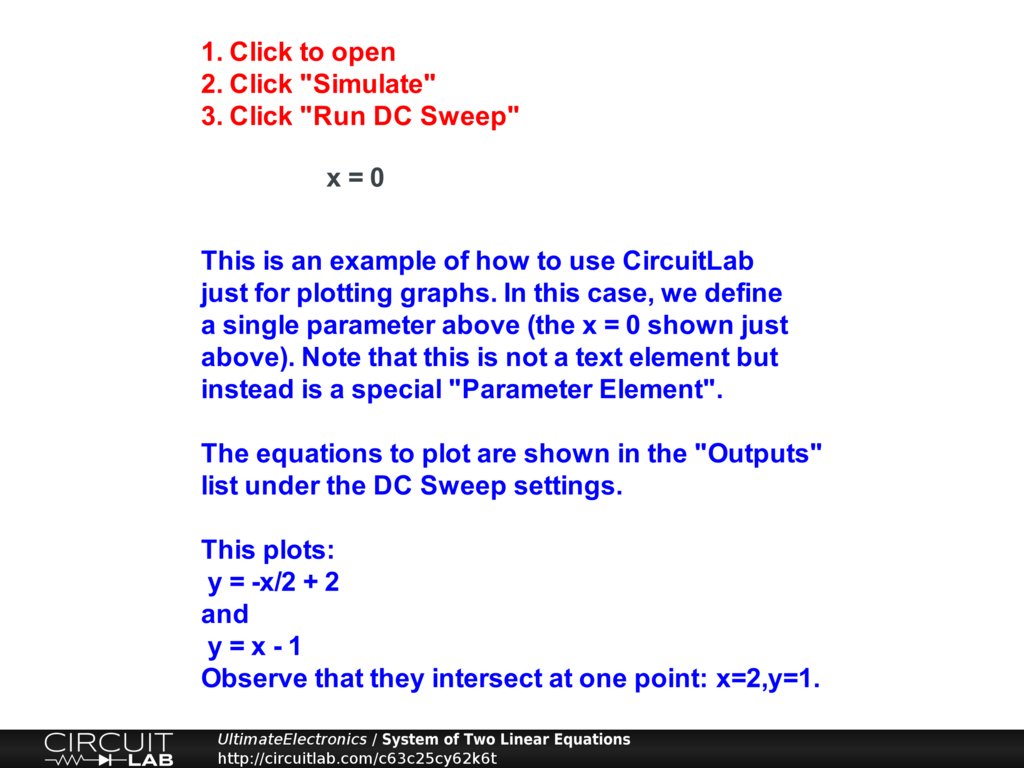System of Two Linear Equations - No Solution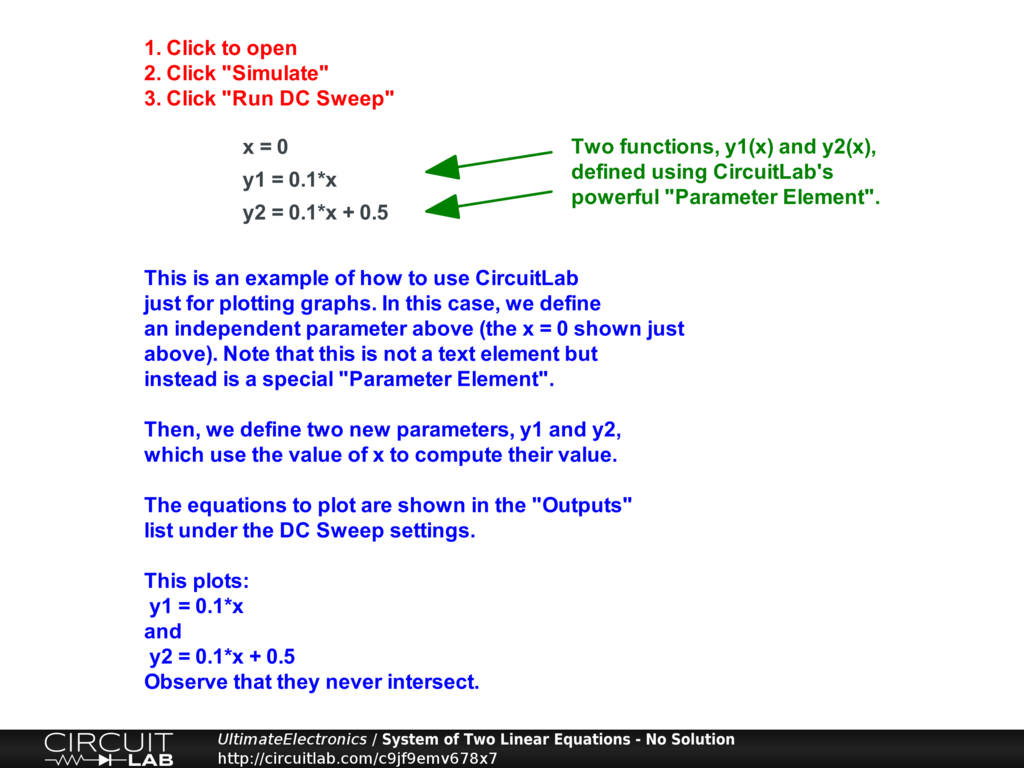Inrush Current Spike when Switching Capacitive Load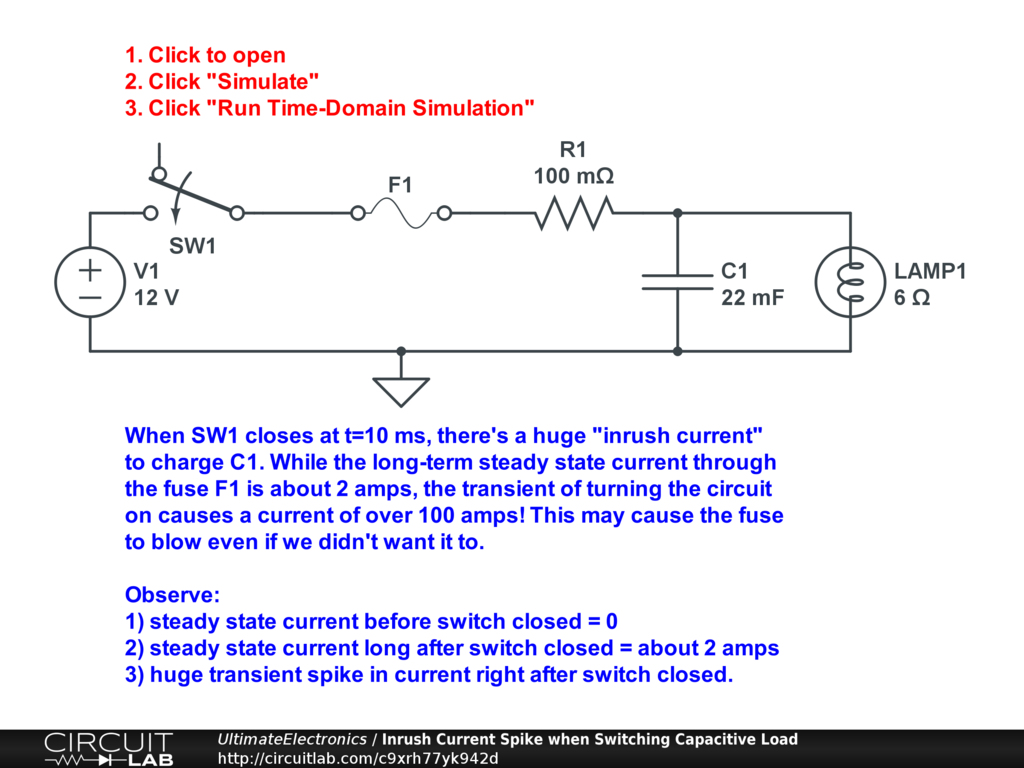From Lumped Element Model:

Lumped Element Model of Spring-Mass-Dashpot Mechanical System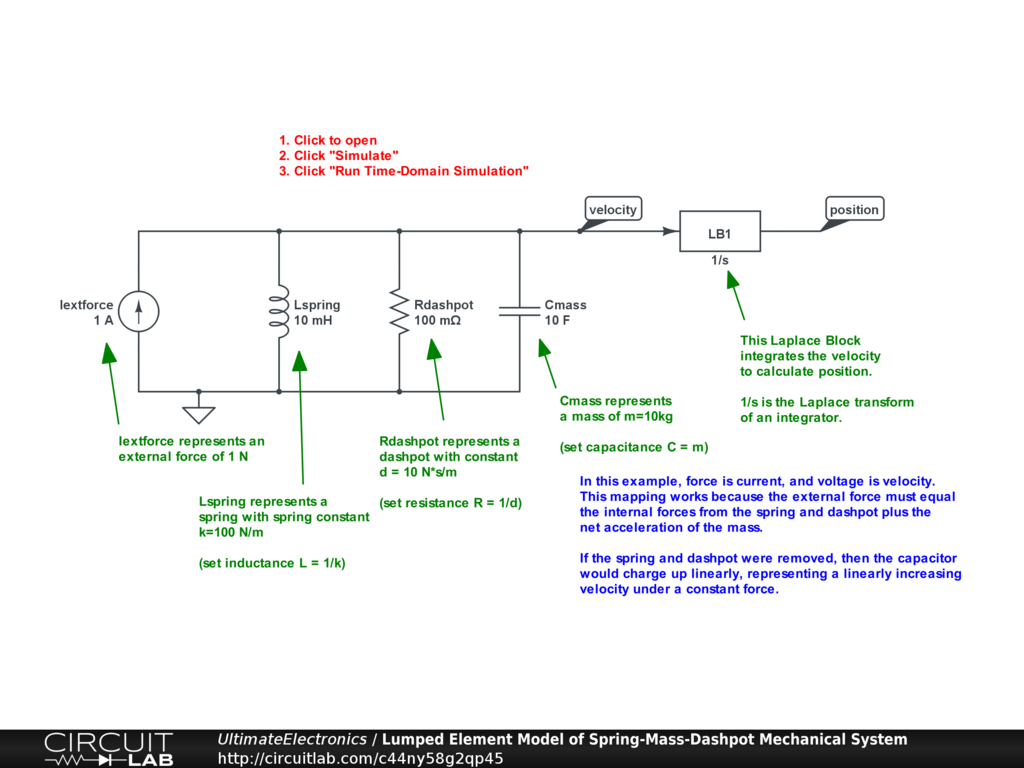Lumped Element Model of Spring-Mass-Dashpot Mechanical System with Laplace Blocks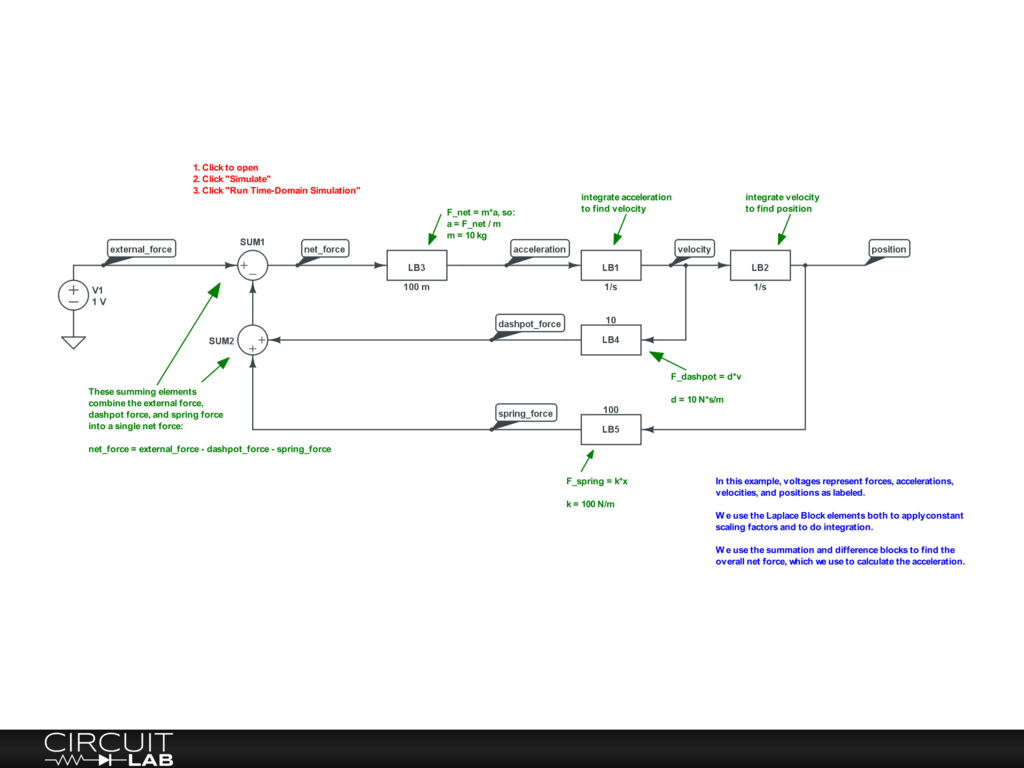Lumped Element Model of Spring-Mass-Dashpot Mechanical System with Laplace Blocks (Parameter Sweep)From Thermodynamics, Energy & Equilibrium:

Thermal Modeling: Electric Hotplate and Skillet Warm-Up